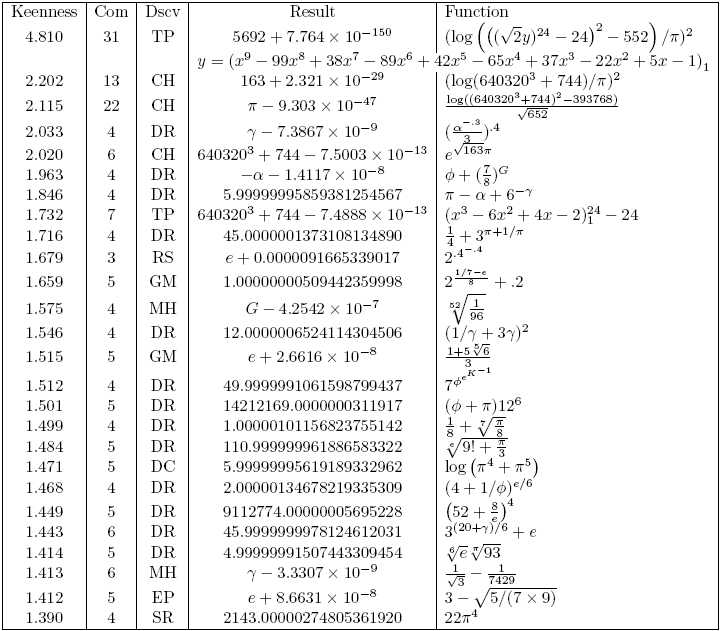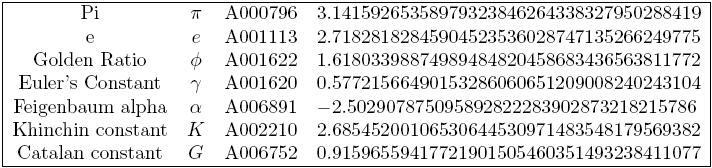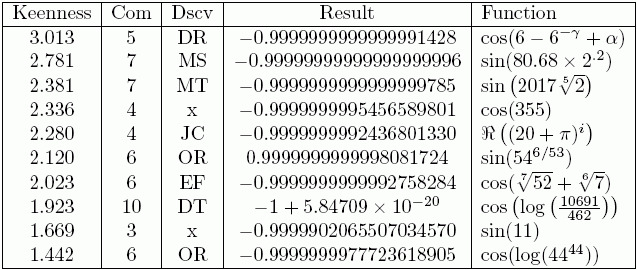## Keen Approximations

Ed Pegg Jr., March 15, 2005

This column generated a lot of good feedback when it appeared last month. I've been given some of the best approximations I've seen.

So what is this word best that I speak of, in a mathematical sense? There are several interesting bestness lists in math:

Best is different from Largest. The largest known prime for the moment is 225,964,951 - 1, but that won't last forever. Likely, it won't even be on the top ten list in 2015, if previous searches are any indication. An example of best -- What is the best packing for 10 circles in a unit square? De Groot proved the best result in 1990. At least four attempts at "best" before him were wrong.

For approximations I wanted something similar. Looking through my thesaurus, I pulled out keenness as a good descriptive term, since approximations need to cut close. I later learned of the Uniformity Conjecture. Daniel Richardson's paper "Testing the Uniformity Conjecture" (postscript, use Ghostview) lists several approximations.

Eric Weisstein has compiled many approximations under Almost Integer, Pi Approximations, Feigenbaum Constant Approximations, Euler-Mascheroni Constant Approximations, e Approximations, Khinchin's Constant Approximations, Catalan's Constant Approximations, and Apéry's Constant Approximations. For the Plouffe Invertor, Simon Plouffe compiled a few approximations of pi. Other lists can be found at Math Magic, Contest Center and Prime Puzzles and Problems Connection. There are a lot of approximations in these pages. Now that I've concocted this concept of keenness, how can I calculate which approximations have the highest keenness?

How should Sin = -0.99999020... be measured? How about the fourth root of 9.1, with a continued fraction that includes 75656, 136181, 3602, 4267, 4841, and 262344 within the first 60 terms? I consideredweird, but nothing particularly special. NJA Sloane found it to be interesting enough to add it to the OEIS as A093876, due to the many unusual large terms.

Did I just say thatwasn't particularly special? That's what I thought when I first wrote this column. Richard Sabey corrected me. 11859210 and 11859211 are the two highest consecutive numbers that are both 19-smooth, that both have all prime factors equal or less than 19. This fact leads directly to.

11859210 ~ 11859211 => 7×13×194 ~ 2×34×5×114 => 91×194 ~ 10×334 => 9.1 ~ 334/194 =>~~ 33/19

So, it turns out thathas an extraordinary continued fraction. For almost all numbers, the geometric mean of the continued fraction approaches Khinchin's constant. The probability that a particular part of the continued fraction is of size s is Log[2, (1 + 1/s)/(1 + 1/(s+1))], or about 1/s2 (Wolfram, p 914). The continued fraction of Sin begins {0, 1, 102108, 14, 3, 1, 1, 3, 1, 1}. Having 102108 as the third term of Sin can be considered unusual.

In asymptotic numbers, on the other hand, it's the norm. For instance, the second term of Zeta is 633825300114114698409864795031, a 30 digit number. The second term of Zeta is a116625189 digit number. Richard Sabey's spectacular approximation to e, (1+9-47*6)3285, gets 18457734525360901453873570 decimal digits of accuracy due to high exponents and asymptotic effects. In objects like π/2-tan-1(1010), the continued fraction begins: {0, 5000000000, 60000000000, 6250000000, 62222222222, 4, 2, 78124999, 1, 1, 3, 1, 62577777777, 6347656250, 62693877551, 48, 1, 2646931, 1, 4, 2, 6, 2, 1, 3002469135, ... }. This behavior is explained through asymptotic series. For high x, π/2-tan-1(x) approaches -1/x + 1/(3*x^3) - 1/(5*x^5) + 1/(7*x^7) - 1/(9*x^9) +...

Continued fractions themselves are asymptotic. The second term in the continued fractions of 355/113 - π is 3748629. The convergents (close fractions) of π are 3, 22/7 (Archimedes), 333/106, 355/113 (Tsu Ch'ung-Chi, 480 AD), 103993/33102, and so on. If you use enough digits, you can get arbitrarily close with a fraction, or with a decimal. 3.1415926535897932385 - π has a second continued fraction term of 26769019461318409709, for example.

Cleverness with functions can cause other problem. Let j = 2, nested under 355 Square Root symbols. Let k = 2, nested under 113 Square Root symbols. Then log2(j)/log2(k)=355/113 -- the same fraction above. Functions cannot be considered free when nesting can produce an infinite number of values.

I've decided keenness = log10(max(CF))/complexity. The top part, log10(max(CF)), is equivalent to the digits of accuracy, and is equal to the number within the first few values of the continued fraction that corresponds to the digits of accuracy. The bottom part, complexity, is equivalent to the digits used.

My rules to measure the keenness of an approximation are as follows:

1. Each digit or constant used adds 1 to the complexity. Each function adds 1 to the complexity.
2. Division, multiplication, addition, subtraction, exponentiation, parenthesization are free. In 1/x, or in x-1, the 1 is free.
3. Asymptotic functions, such as Zeta or Cot, are not allowed, nor are Pisot numbers.
4. Root objects, such as Pi ~ {1+x+6x2+x3-5x4-5x5+2x6}2 can be thought of as {1,1,6,1,-5,-5,2}2 (complexity 8). Zeros count.
5. Sin and Cos are not allowed, since they can be used to arbitrarily double keenness.

The approximations with the highest keenness are as follows (TeX):CH is Charles Hermite, DC is Dario Castellanos, DR is Derek Ross, DT is David Terr, EF is Erich Friedman, EP is Ed Pegg Jr, GM is Geoffrey Morley, JC is John H. Conway, MH is Mark Hudson, MS is Mike Shafer, OR is Oliver Runge, RS is Richard Sabey, SR is Srinivasa Ramanujan, and TP is Titus Piezas III. The constants used here are as follows:Here are some notes on some of these:

4.810: Based on 1423, which has class number 9, seems to stand out in the wonderful analysis by Titus Piezas III, On Keen Approximations. This might be hard upper limit for class-number related polynomials, as he notes in his article.

2.202, 2.115, 2.020, 1.732: These are variations of very deep mathematics. The simple polynomial x3 - 6x2 + 4x - 2, sparked my interest in this topic. I first learned of it in Titus Piezas' article, Ramanujan’s Constant (eπ√163) And Its Cousins. More of the mathematics involved can be seen through the j-Function, the Ramanujan Constant, and the Pisot numbers. See also On Keen Approximations for other varieties.

• 262537412640768743.9999999999992500726 is eπ√163 -- a result of the j-Function
• 262537412640768743.9999999999992511239 is (x3 - 6x2 + 4x - 2)24 - 24. (The "-24" isn't counted in the complexity.)
• 262537412640768744 is 6403203 + 744.
• j(q) = 1/q + 744 + (1 + 196883)q + (1+196883+21296876)q2 + (2×1+2×196883+21296876+842609326)q3 -- the j-Function
• 1, 196883, 21296876, 842609326, 18538750076 -- A001379 -- Degrees of irreducible representations of Monster group M
• x11+2x10-x9-x7-x6-x5+2x4+x-1 (mod 4) = g4(x). With zerosum check, a self-dual code of length 24. Generates the Leech Lattice.

The Leech Lattice, connected to perfect hypersphere-packings in the 24th dimension, is seemingly connected to (x3 - 6x2 + 4x - 2)24 - 24 and eπ√163. When John McKay noticed (1+196883) from the Griess algebra and from the j-function, John Conway initially dismissed it as "moonshine." The connection was proven in 1992 by Richard Borcherds via the no-ghost theorem from string theory, which garnered him the Fields Medal, the highest honor in Mathematics.

I stripped out results using sin() and cos() from the original table. The reason sin(11) works well is because 22/7 (3.142857) is an approximation to π (3.141592). It takes the closeness of 11 to 7π/2 and squares it. All of these use a π approximation of some sort. If you take a result from the above table, the apparent keenness can be almost doubled.If you can find other approximations with a keenness above 1.4, I'd love to hear from you (ed@mathpuzzle.com). I will maintain this list and hone the rules until a really solid top twenty list is produced.

References:

John Conway and Neil Sloane, Sphere Packings, Lattices, and Groups, Springer 1999.

Erich Friedman, Math Magic August 2004: Approximations. http://www.stetson.edu/%7Eefriedma/mathmagic/0804.html.

Abderrahmane Nitaj, "The ABC Conjecture Home Page," http://www.math.unicaen.fr/~nitaj/abc.html.

Titus Piezas, Ramanujan’s Constant (eπ√163) And Its Cousins. http://www.geocities.com/titus_piezas/Ramanujan_a.htm.

Simon Plouffe, Plouffe's Invertor, http://pi.lacim.uqam.ca/eng/.

Carlos Rivera, "The best approximation to pi with primes," http://www.primepuzzles.net/puzzles/puzz_050.htm.

Stephen Wolfram, A New Kind of Science, Wolfram Media, 2002, page 914. http://www.wolframscience.com/nksonline/page-914d-text.

Math Games archives.

Comments are welcome. Please send comments to Ed Pegg Jr. at ed@mathpuzzle.com.

Ed Pegg Jr. is the webmaster for mathpuzzle.com. He works at Wolfram Research, Inc. as an associate editor of MathWorld, and as administrator of the Mathematica Information Center.